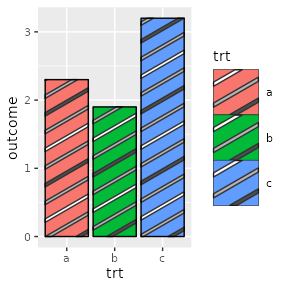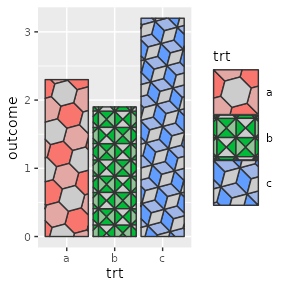# Developing Patterns

library("ggplot2")
library("ggpattern")

## Developing Patterns - overview

To develop a pattern for use with {ggpattern} (and any other packages that use {gridpattern}) you will need to:

1. Decide whether this is a geometry-based pattern or an array-based pattern.
2. Create a function with the required arguments and return value
3. Use options() to let {gridpattern} know that a certain pattern name should be delegated to your function.

This vignette shows how to:

• write a create_pattern() function of the correct signature for a geometry-based pattern or array-based pattern
• Instruct {ggpattern} on where to find this user-defined pattern

## Pattern Classification - geometry-based and array-based

There are only 2 high-level classes of pattern supported by gridpattern - geometry-based and array-based.

Geometry-based patterns create a series of geometry objects and trim them (using sf::st_intersection(), gridGeometry::polyclipGrob(), gridpattern::alphaMaskGrob(), etc) to be within the boundary of the grob. For example, the ‘stripes’ pattern in gridpattern is a series of equally spaced rectangular polygons.

Array-based patterns are RGBA image arrays. Any supplied image will be processed by gridpattern to ensure it is masked to only apply to the area within the grob’s boundary.

## {ggpattern} Aesthetics - Descriptions and Defaults

Although custom {gridpattern} pattern parameters need not limit itself to the set of aesthetics provided by {ggpattern} doing so may make your pattern more useful for others:

{ggpattern} aesthetic summary - click to open/close
aesthetic description default possible values
pattern Name of the pattern to draw ‘stripe’ gridpattern::names_pattern
pattern_alpha Alpha 1 value in range [0, 1] or NA
pattern_angle Rotation angle (entire pattern) 30 angle in degrees
pattern_aspect_ratio Aspect ratio adjustment NA usual range [0.01, 10]
pattern_colour Stroke colour ‘grey20’ colour
pattern_density Approx. fraction of area the pattern fills 0.2 value in range [0, 1]
pattern_filename Image filename/URL ’’ Filename/URL
pattern_fill2 Second fill colour ‘#4169E1’ colour
pattern_fill Fill colour ‘grey80’ colour
pattern_filter Image scaling filter ‘lanczos’ magick::filter_types
pattern_frequency Frequency 0.1
pattern_gravity Image placement ‘center’ magick::gravity_types
pattern_grid Pattern grid type ‘square’ ‘square’, ‘hex’, ‘hex_circle’
pattern_key_scale_factor Scale factor for pattern in legend 1
pattern_linetype Stroke linetype 1 linetype
pattern_option_1 - 5 Generic options for expansion 0
pattern_orientation Orientation ‘vertical’ ‘vertical’, ‘horizontal’, ‘radial’
pattern_phase Phase 0
pattern_res Pattern resolution (pixels per inch) NA
pattern_rot Rotation angle (shape within pattern) 0 angle in degrees
pattern_scale Scale 1 Multiplier
pattern_shape Plotting shape 1 shapes
pattern_size Stroke linewidth 1 linewidth
pattern_spacing Spacing between repetitions of pattern 0.05 value in range [0, 1] (snpc units)
pattern_subtype Generic control option NA pattern-dependent
pattern_type Generic control option NA pattern-dependent
pattern_xoffset Shift pattern along x axis 0 value in range [0, 1] (snpc units)
pattern_yoffset Shift pattern along y axis 0 value in range [0, 1] (snpc units)

Note {ggpattern} may also pass other geom aesthetics of possible interest such as fill. Also note that {ggpattern} will only pass pattern aesthetics values of length one but if the pattern is called directly by gridpattern::patternGrob() then the pattern may be passed pattern parameters of arbitrary length.

## Geometry-based pattern functions - Formal Arguments and Return Values

All geometry-based pattern creation functions must:

1. Have the exact function signature: function(params, boundary_df, aspect_ratio, legend)

• params - parameters for the pattern (the aesthetics) e.g pattern_fill
• boundary_df - data.frame containing polygon information i.e. The polygon_df format.
• aspect_ratio - the best guess that {gridpattern} / {ggpattern} is able to make as to the aspect ratio of the viewport in which this pattern is being rendered.
• legend logical value to indicate whether or not this function is being called to render a key legend or the in-place geom fill.
2. Return a grid grob object. This can be any valid grob including a grid::grobTree(). The user should make sure it lies within the boundary represented by boundary_df either by clipping with functions like sf::st_intersection(), gridGeometry::polyclipGrob(), gridpattern::alphaMaskGrob(), etc. or using bounded grob functions like gridpattern::patternGrob() or grid::polygonGrob().

## Array-based pattern functions - Formal Arguments and Return Values

All array-based pattern creation functions must:

1. Have the exact function signature: function(width, height, params, legend)
• width,height - dimensions of the bounding box of the geom area
• params - parameters from the geom (the aesthetics) e.g pattern_fill
• legend logical value to indicate whether or not this function is being called to render a key legend or the in-place geom fill.
2. Return a 3D array of RGBA values (all values in the range [0, 1]). gridpattern itself will mask this image so that it only applies to the area within the grob’s boundary.

## The polygon_df data.frame format

The polygon_df is a very simple data.frame format to contain polygon values. This is used to pass the coordinates of the geom boundary from the geom to the pattern generating function.

It contains only ‘x’ and ‘y’ columns for the coordinates, and an ‘id’ column used to signify which polygon the coordinates belong to.

The following polygon_df data.frame contains 2 polygons:

example data in ‘polygon_df’ format
x y id
0 0 1
1 0 1
1 1 1
0 1 1
0 0 2
2 0 2
2 1 2
0 1 2

## Associating a function with {gridpattern} pattern name

There are two global option() values which can be set - one for geometry-based patterns, and the other for array-based patterns.

The global values should point to a named list, where the names are the pattern names you want to use within {gridpattern}, and the named values are the actual functions. Note for backwards-compatibility with the original {ggpattern} system these options start with ggpattern instead of gridpattern.

options(ggpattern_array_funcs    = list(your_pattern_name = your_pattern_function))
options(ggpattern_geometry_funcs = list(your_pattern_name = your_pattern_function))

Pattern names must be different from any of the builtin patterns included in {gridpattern}.

## Example: Multiple colored stripes (serializing aesthetics)

All geometry-based pattern creation functions must:

1. Have the exact function signature: function(params, boundary_df, aspect_ratio, legend)
• params - parameters from the geom (the aesthetics) e.g pattern_fill
• boundary_df - data.frame containing polygon information i.e. The polygon_df format.
• aspect_ratio - the best guess that {gridpattern} / {ggpattern} is able to make as to the aspect ratio of the viewport in which this pattern is being rendered.
• legend logical value to indicate whether or not this function is being called to render a key legend or the in-place geom fill.
2. Return a grid grob bounded by the boundary represented by boundary_df (including grid structures like a grid::grobTree()).

Since {ggplot2} only allows single-valued aesthetics you can only normally create single-colored stripe patterns in {ggpattern} even though the underlying {gridpattern} stripe pattern supports multiple-colored stripes. In this example we’ll create a ‘multicolor_stripe’ pattern that serializes multiple pattern_colour and pattern_fill colors using comma-separated values.

multicolor_stripe_pattern <- function(params, boundary_df, aspect_ratio,
legend = FALSE) {
args <- as.list(params)
args <- args[grep("^pattern_", names(args))]

args$pattern_colour <- strsplit(args$pattern_colour, ",")[]
args$pattern_fill <- strsplit(args$pattern_fill, ",")[]

args$pattern <- "stripe" args$x <- boundary_df$x args$y <- boundary_df$y args$id <- boundary_df$id args$prefix <- ""

do.call(gridpattern::patternGrob, args)
}

A global option ggpattern_geometry_funcs is a named list which contains geometry-based pattern creating functions to use outside of ggpattern.

The name used in this list corresponds to the pattern name used with the geom - in this case we will be using pattern = 'polygon'.

options(ggpattern_geometry_funcs = list(multicolor_stripe = multicolor_stripe_pattern))
df <- data.frame(trt = c("a", "b", "c"), outcome = c(2.3, 1.9, 3.2))
ggplot(df, aes(trt, outcome)) +
geom_col_pattern(aes(fill = trt), colour = 'black',
pattern = 'multicolor_stripe',
pattern_fill = "grey30,grey70,white,grey70",
pattern_density = 0.2,
pattern_spacing = 0.1) +
theme(legend.key.size = unit(1.2, 'cm'))## Example: Three-color polygon tilings (adapting {ggplot2} aesthetics)

All geometry-based pattern creation functions must:

1. Have the exact function signature: function(params, boundary_df, aspect_ratio, legend)
• params - parameters from the geom (the aesthetics) e.g pattern_fill
• boundary_df - data.frame containing polygon information i.e. The polygon_df format.
• aspect_ratio - the best guess that {gridpattern} / {ggpattern} is able to make as to the aspect ratio of the viewport in which this pattern is being rendered.
• legend logical value to indicate whether or not this function is being called to render a key legend or the in-place geom fill.
2. Return a grid grob bounded by the boundary represented by boundary_df (including grid structures like a grid::grobTree()).

For this example we’ll adapt the built-in ‘polygon_tiling’ pattern to create a new ‘tiling3’ pattern that fills the polygon tiling with three colors: the fill aesthetic, the pattern_fill aesthetic, and their “average” color.

tiling3_pattern <- function(params, boundary_df, aspect_ratio, legend = FALSE) {
args <- as.list(params)
args <- args[grep("^pattern_", names(args))]

# hexagonal tiling using "regular_polygon" pattern
args$pattern <- "polygon_tiling" # three-color tiling using fill, pattern_fill and their "average" avg_col <- gridpattern::mean_col(params$fill, params$pattern_fill) args$pattern_fill <- c(params$fill, avg_col, args$pattern_fill)

args$x <- boundary_df$x
args$y <- boundary_df$y
args$id <- boundary_df$id
args\$prefix <- ""

do.call(gridpattern::patternGrob, args)
}

A global option ggpattern_geometry_funcs is a named list which contains geometry-based pattern creating functions to use outside of ggpattern.

The name used in this list corresponds to the pattern name used with the geom - in this case we will be using pattern = 'complex'.

options(ggpattern_geometry_funcs = list(tiling3 = tiling3_pattern))
df <- data.frame(trt = c("a", "b", "c"), outcome = c(2.3, 1.9, 3.2))
ggplot(df, aes(trt, outcome)) +
geom_col_pattern(aes(fill = trt, pattern_type = trt),
pattern = 'tiling3',
pattern_angle = 45,
pattern_spacing = 0.15) +
scale_pattern_type_manual(values = c("hexagonal", "tetrakis_square", "rhombille")) +
theme(legend.key.size = unit(1.2, 'cm'))## Other examples

The {gridpattern} package contains a vignette on developing {gridpattern} / {ggpattern} patterns with a few more examples including an example of layering patterns on top of each other. Use vignette("developing-patterns", package="gridpattern") to see it.

Also the {gridpattern} source contains the full source code for seventeen patterns: https://github.com/trevorld/gridpattern/tree/main/R